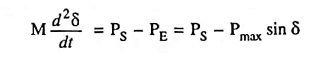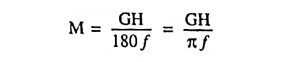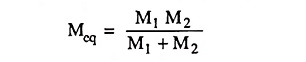## Power System Stability Interview Questions and Answers:

1. Define stability limit.

Ans. A stability limit is the maximum power flow possible through some particular point in the system or the part of the system to which the stability limit refers is operating with stability.

Ans. Steady state stability may be defined as the capability of an electric power system to maintain synchronism between machines within the system and external tie lines following a small slow disturbance (normal load fluctuations, the actions of automatic voltage regulators and turbine governors).

3. What is meant by steady state stability limit ?

Ans. The steady state stability limit refers to the maximum power which can be transferred through the system without loss of stability.

4. Distinguish between steady state stability and transient stability of a power system.

Ans. Steady state stability refers to the conditions of stability in response to small and gradual changes of load while transient stability refers to the conditions of stability in response to large sudden disturbances. A sudden large disturbance includes application of faults, clearing of faults, sudden load changes, and inadvertent tripping of lines and generators.

5. Define dynamic stability of a power system.

Ans. Dynamic stability is the ability of a power system to remain in synchronism after the initial swing (transient stability period) until the system has settled down to the new steady state equilibrium condition.

6. Define load angle of a generator.

Ans. Angle between rotor pole axis and stator rotating field axis (both rotating together at synchronous speed) is called the load angle. It is expressed in electrical degrees or electrical radians.

7. What is meant by power angle curve ?

Ans. Power angle curve is a curve of power transfer P versus power angle δ. It is a sine curve.

8. What is meant by a swing curve ?

Ans. Swing curve is a graph of power angle δ versus time t.

9. On what basis do you conclude that a given synchronous machine has lost stability ?

Ans. In case the power angle δ reduces after attaining maxima corresponding dδ/dt = 0 condition indicates stability. On contrary if the power angle exceeds critical angle of load, the system becomes unstable.

10. Define infinite bus in a power system.

Ans. Infinite bus has constant voltage, constant electrical angle of the voltage, constant frequency, infinite fault level and, therefore, the quantities of infinite bus do not get affected by connecting or disconnecting individual machines or transmission lines.

11. How can steady state limit be improved ?

Ans. Steady state limit can be improved either by increasing the excitation of the machine/machines or by reducing transfer reactance.

12. Write swing equation of a single machine.

Ans. The equation giving the relative motion of rotor (load angle δ) with respect to the stator field as a function of time is called the swing equation.

The swing equation for a single-machine system can be written aswhere M is angular momentum, PS is shaft power (mechanical power), PE is electromagnetic power, δ is load angle between E and V and Pmax is the maximum steady state power.

13. Define inertia constants M and H.

Ans. M is the angular momentum of the machine and is expressed in joule-seconds per radian or in mega-joule-seconds per electrical degree. M, the inertia constant of the machine, depends on the type and size of the machine.

Another constant H having narrow range of values for each class of machine regardless of its capacity is defined as the ratio of the kinetic energy stored at rated speed to the rated power of the machine. H is expressed in MJ/MVA.

14. Give typical values of inertia constant H for turbogenerators and slow-speed waterwheel generators.

Ans. The average value of H in MJ/MVA for turbogenerators is between 3 and 9 and for waterwheel generators it is between 2 and 4.

15. Write down the units of inertia constants M and H and their interrelationships.

Ans. M is expressed in MJ-seconds/electrical degree or MJ-seconds/electrical radian and H is expressed in MJ/MVA.

The interrelationship between M and H is given as16. What is equal area criterion ?

Ans. Equal area criterion is a simple graphical method of predicting the transient stability of two-machine systems or a single machine against infinite bus. This criterion does not require solution of swing equation for determination of stability conditions.

The stability conditions are determined by equating the areas of segments on the power angle diagram between the P-curve and the new power transfer line for the given condition.

17. If the two machines with inertias M1 and M2 are swinging together, what will be the inertia of the equivalent machine ?

Ans. Equivalent machine inertia is given as18. Sketch typical swing curves for a synchronous machine: (i) showing that the machine is stable after a disturbance. (ii) showing that the machine is unstable after a disturbance.

Ans. If the system is unstable, δ(t) continues to increase indefinitely with time and the machine loses synchronism.

On the other hand, if the system is stable, δ(t) performs oscillations (nonsinusoidal) whose amplitude decreases in actual practice owing to damping terms.

Scroll to Top